# AP Calculus BC Practice Test 4

### Test Information9 questions27 minutes

Calculator Allowed

1. The first three non-zero terms in the Taylor series about x = 0 for f(x) = cos x are

2.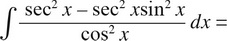3.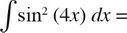4. To what limit does the sequence Sn =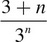converge as n approaches infinity?

5.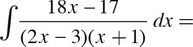6. A particle moves along a path described by x = cos3t and y = sin3t. The distance that the particle travels along the path from t = 0 to t =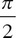is

7. The sale price of an item is 800 - 35x dollars and the total manufacturing cost is 2x3 - 140x2 + 2,600x + 10,000 dollars, where x is the number of items. What number of items should be manufactured in order to optimize the manufacturer’s total profit?

8. The area enclosed by the polar equation r = 4 + cos θ, for 0 ≤ θ ≤ 2π, is

9. Use the trapezoid rule with n = 4 to approximate the area between the curve y = x3 - x2 and the x-axis from x = 3 to x = 4.